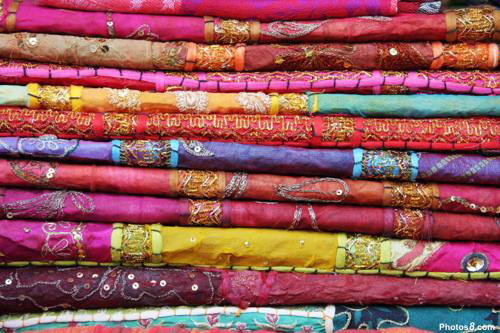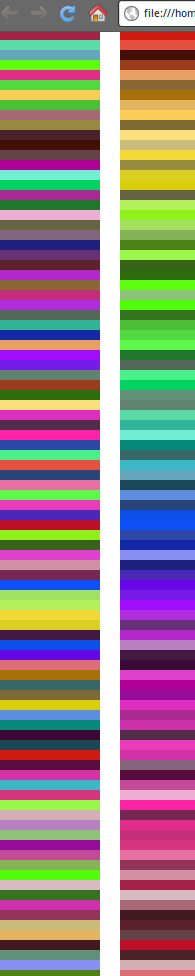I was recently tasked with creating a color pallete for some custom (legacy) code for an existing e-commerce website. One of the requirements was to show the colors grouped by similar tint. I thought to myself, this is a classic sorting problem. There was a catch, though. What do we sort?

## Converting from HTML Hex to HSV

Turns out, before we can do any sort of sorting, we need to convert the HTML Hex value of a color to HSV (Hue Saturation Value) format. A more detailed description of HSV can be found here: http://en.wikipedia.org/wiki/HSL_and_HSV.

To convert hex to HSV, we first have to get the RGB values. This is really easy to do.

The code is simple. Each RGB value corresponds to a two character hex code in the hex string. So we use parseInt() to get the value of the substring. We pass 16 to parseInt() to make sure it is parsed as a hex value.

Now that we have the RGB values of the hex, we can calculate the Chroma. The Chroma will be used to calculate the Hue and Saturation. From the wiki:

M = max(R,G,B)

m = min(R,G,B)

Chr = M - m

Simple enough. By default, our Hue and Saturation are 0. Both depend on the Value. In HSV format, the Value is simply the max of the RGB values:

Getting the min and max of the RGB values was easy using the built-in Math functionality of JavaScript. I got this directly from John Resig: http://ejohn.org/blog/fast-javascript-maxmin/.

Now, we proceed to Saturation and Hue. Depending on Saturation, we may never have to calculate the Hue. So, we should check and make sure Saturation is greater than 0. According to the wiki:

Sat = Chr/V where V is the Value

If Saturation ends up being 0, the color is somewhere between white and black (some sort of gray), so Hue will be 0. If Saturation is greater than 0, the color has some sort of tint. The Hue must be calculated. The Hue is explained very well in the wiki with diagrams. Here are the formulas:

if R is Max: Hue = (((G-min)-(B-min))/Chr)*60

if G is Max: Hue = 120+(((B-min)-(R-min))/Chr)*60

if B is Max: Hue = 240+(((R-min)-(G-min))/Chr)*60

So to get the Saturation and Hue, here is the code:

Simple enough, right? Note, that in the case where R is the Max, we must account for any wraparound 360 degrees.

## Sorting by Hue

Now that we can convert HTML Hex colors to HSV, we can group similar tinted colors together. We basically sort the hex colors by Hue!

Using the code samples above, we can create a `sortColors()` JavaScript function that will take an existing array of HTML Hex Color objects, find the HSV value for each, and sort them according the Hue. For simplicity’s sake, the following code will modify the existing array by adding the Hue, Saturation, and Value to each object.

We take advantage of JavaScript Array sorting to return the array sorted by Hue. This is better explained by W3C here: http://www.w3schools.com/jsref/jsref_sort.asp.

## What about Saturation and Value?

### Value

Though sorting by Hue might be good enough in most cases, sometimes you want the pallette to look even more precise. You can sort the array further by sorting by Value within each of the different Hues. Each of the different Hues lie within 60 degrees of each other (0 to 60, 61 to 120, 121 to 180, etc.).

### Saturation

If you would like to pull out white, black, and all gray colors, you will want to remove any colors with a saturation close to 0. I’ve found that a Saturation < .09 will usually be a safe condition to use.

## An Example using `jQuery.tmpl()` and `sortColors()`

Here is a screenshot of the results of `sortColors()` displayed using jQuery templates.Unfortunately, Blogger does not do file hosting, so I can’t upload the example code. But shoot me an email and I’ll be glad to send it over.

## Resources:

jQuery.tmpl()

RGB to HSV in C

HSL and HSV Wiki

Fast JavaScript Max/Min

Random HTML Color Generator